﻿ Generating Random Colors

Color

Background

Done

# Generating Random Colors

by kirupa   |   filed under JavaScript 101

Give your apps some flair by learning how to generate random colors...both RGB and HSLA, like a pro!

When it comes to colors, most of the time your choice on what color to use will be deliberate. You may have a style guide you have to follow. Your design team may have provided feedback on a particular color that makes the most sense. Maybe, you just like this shade of green and, against the wishes all your more artistically talented friends, want to use it in a lot of places: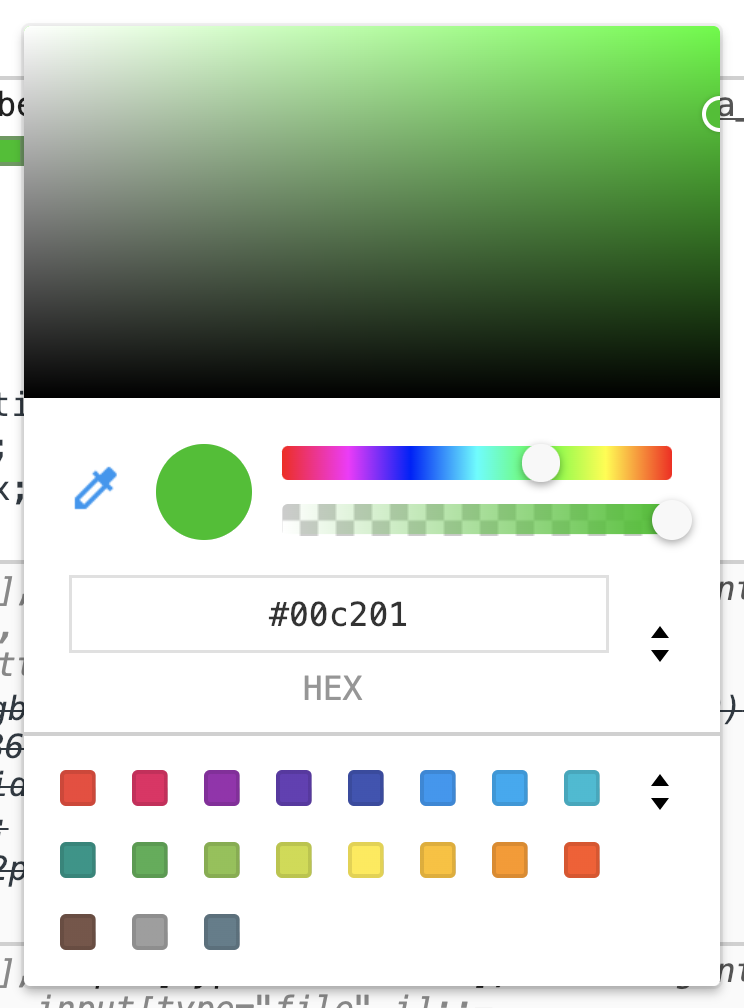Now, there will be times when there is no single color that you need to use. You could be creating an effect where your app cycles through different colors and themes of colors. You could be in a situation where the best color is one that is randomly chosen for you. This article is going to prepare you for such a situation. In this article, we are going to look at how you can generate random colors, and we will do this looking across two approaches. One approach will be simple but limiting. Another approach will be more complex but highly customizable. What are we waiting for?

Onwards!

## The Simple Approach

If all you want to do is generate a random color, and you don’t really care about anything else, then the following code is for you:

``````function getRandomColor() {
let characters = "0123456789ABCDEF";
let color = '#';

for (let i = 0; i < 6; i++) {
color += characters[getRandomNumber(0, 15)];
}

return color;
}

function getRandomNumber(low, high) {
let r = Math.floor(Math.random() * (high - low + 1)) + low;
return r;
}``````

All you need to do is call the getRandomColor function. This function will return a randomly generated hex value that you can use wherever fine color values are accepted. Below is a snippet of us using it to set the background color of an element referenced by square:

``````let color = getRandomColor();
square.style.backgroundColor = color;``````

To see this in action, take a look at the following animation:

You will see a square changing color every few seconds to a different randomly generated color. You can take a look at the full source code for this here to see how it all gets tied together.

#### How this code works!

The way this code works is surprisingly direct. We know a hex color value is made up of six characters with each character being anything from 0 to F. All we are doing is randomly selecting a character from 0123456789ABCDEF and generating our color value by repeating this process six times. Pretty direct indeed, right?

## The Customizable Approach

Sometimes, you still want to generate random colors, but you want to have some control over just how random the colors that get generated are. Using the simple approach we saw earlier, we are at the mercy of what random numbers get called to generate the final color. For some of you, that is acceptable. If that doesn't seem very appealing, then we have this slightly more involved solution that relies on the HSL color format.

If you are not familiar with HSL or its transparency-friendly HSLA variant, it is a widely supported color format that allows you to specify four parts that feed into the color you ultimately see: hue, saturation, lightness, alpha. While going into detail about HSLA goes beyond the scope of what we are doing here, we will look at their four parts very briefly to help us generate the exact range of random colors we want.

### Hue

Hue is the actual color you are going for, and it is represented in terms of a color wheel: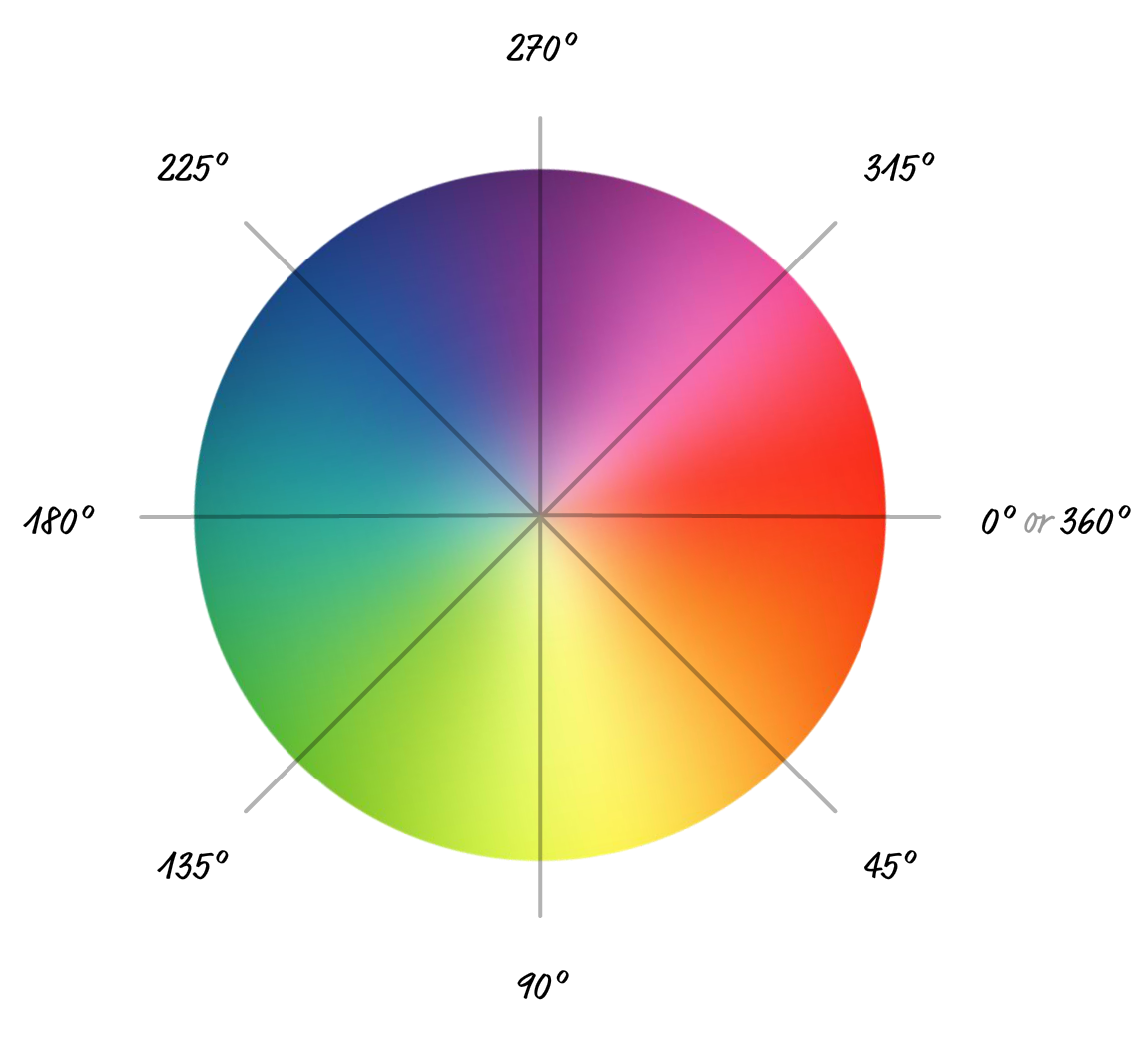Any color you want is represented by a degree value that goes from 0 to 360 with red at the 0 degree mark, green at 120, and blue at 240.

### Saturation

Saturation refers to how intense the color appears, and it goes from a value of 0 to 100: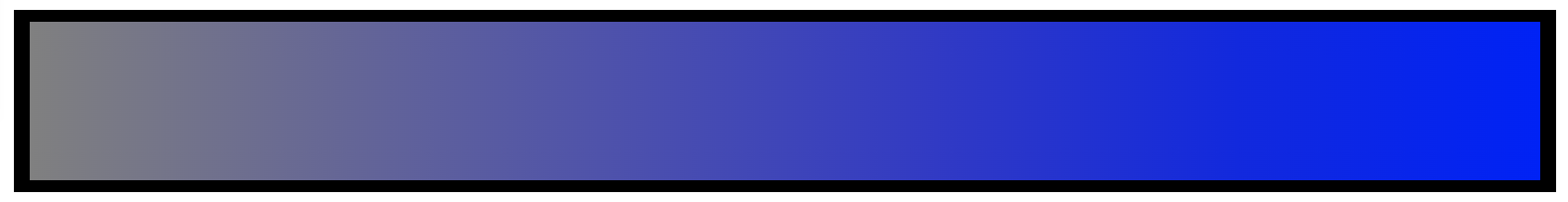A value 0 is almost no intensity, which results in a final output of gray. When you go with 100, the fullness of the hue you picked earlier shows through with maximum intensity.

### Lightness

The next value you specify is something known as lightness. Imagine you have a bright white light that you can shine onto a color. A value of 0 means that none of that light shines onto our color. It is the equivalent of complete darkness. A value of 100 means the full wrath of that light shines onto our color to simulate complete brightness:If you aren't careful, your color could end up appearing fully black or fully white.

### Alpha

The alpha value describes how transparent our color will be. A value of 0 is full transparency, and value of 1 is full opacity: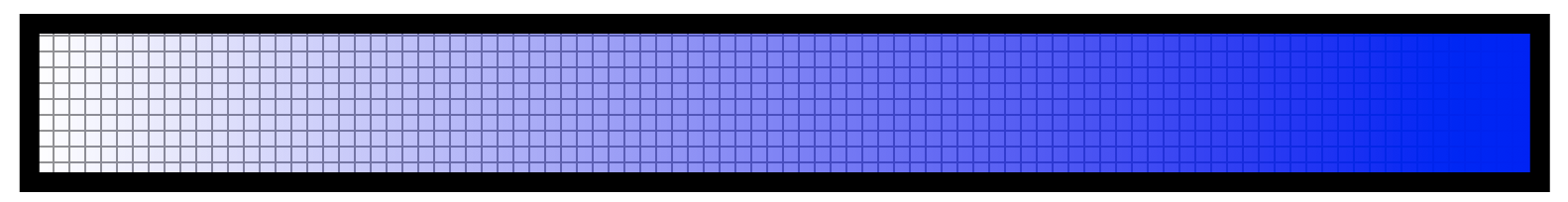If you have set the opacity property in CSS before, the value you specify here is identical in behavior to that. While specifying the alpha is optional, for our purposes, we will explicitly specify it.

### Putting it All Together

When you take the hue, saturation, lightness, and alpha values, the HSLA output takes the following form: hsla(hue, saturation, lightness, alpha). If we used some real values for a blue color (hue of 240) that is fairly intense (saturation of 75), nearly fully illuminated (lightness of 90), and has no transparency (alpha of 1), you will get the following value: hsla(240, 75, 90, 1). This value will be in the form of a string that our CSS and JavaScript know what to do with when paired with a property that expects a color value. Our goal is to generate that string sequence whose numerical values will be within a range of random numbers that we specify.

### The Code

There is a reason why we got a quick overview of the values that make up a HSLA color, and that is because we are going to specify our own range of values for each one as part of constraining how random the final color that gets generated actually will be. That is much simpler than the previous, really long sentence makes it out to be.

First, let's look at the code that we will use for generating our customizable random color:

``````function getRandomColor(h, s, l, a) {
let hue = getRandomNumber(h, h);
let saturation = getRandomNumber(s, s);
let lightness = getRandomNumber(l, l);
let alpha = getRandomNumber(a * 100, a * 100) / 100;

return {
h: hue,
s: saturation,
l: lightness,
a: alpha,
hslaValue: getHSLAColor(hue, saturation, lightness, alpha)
}
}

function getRandomNumber(low, high) {
let r = Math.floor(Math.random() * (high - low + 1)) + low;
return r;
}

function getHSLAColor(h, s, l, a) {
return `hsl(\${h}, \${s}%, \${l}%, \${a})`;
}``````

The entry point to generating our random color is the getRandomColor function, but simply calling getRandomColor isn't enough like in our simple case earlier.

In this variation of getRandomColor, We pass in four arguments that correspond to h, s, l, and a. Sounds simple enough, but wait, there is more! The arguments aren't a single value. Instead they each specify a minimum and maximum range we want the values to randomly fall in-between, and we specify this range by using an array. All of this is much simpler than it sounds, and here is what the code to call getRandomColor looks like:

``````let h_range = [0, 360];
let s_range = [90, 100];
let l_range = [0, 90];
let a_range = [1, 1];

let color = getRandomColor(h_range, s_range, l_range, a_range);``````

Notice the range of numbers we have specified. The random value returned by getRandomColor for each argument will fall somewhere within the specified range. Now, if you don't want to specify each range as its own variable, you can totally just specify the range as part of the argument directly:

``````let color = getRandomColor([0, 360],
[90, 100],
[0, 90],
[1, 1]);``````

There is no right or wrong way to do this as long as you specify the range you care about. Anyway, using these arguments, the ranges are as follows: the random value for hue will be between 0 and 360, 90 and 100 for saturation, 0 and 90 for lightness, and between 1 and...1 for alpha. By specifying the same value for the minimum and maximum of our range like we do for alpha, we ensure that only that value gets returned. It's a simple way to force one value without any randomness interfering, so keep that trick in mind.

Here is the part that you really care about. What gets returned by getRandomColor is an object containing five properties, which can easily be seen whe we look at the code:

``````function getRandomColor(h, s, l, a) {
let hue = getRandomNumber(h, h);
let saturation = getRandomNumber(s, s);
let lightness = getRandomNumber(l, l);
let alpha = getRandomNumber(a * 100, a * 100) / 100;

return {
h: hue,
s: saturation,
l: lightness,
a: alpha,
hslaValue: getHSLAColor(hue, saturation, lightness, alpha)
}
}``````

On the returned object, the h, s, l, and a properties return the corresponding randomly generated value based on the range we passed in as an argument. The hlsaValue property returns the final string (ie: hsla(240, 75, 90, 1)) that we can pass directly to any property in CSS or JavaScript that expects a color value.

Below is an example that shows all of this working:

``````let h_range = [0, 360];
let s_range = [90, 100];
let l_range = [0, 90];
let a_range = [1, 1];

let color = getRandomColor(h_range, s_range, l_range, a_range);
square.style.backgroundColor = color.hslaValue;``````

We have an element called square and we are setting its background color to the randomly generated HSLA value. The following animation uses the above snippet to change the square's color (view source):

The final color will respect the range values you specified earlier, and any tweaks you make to the ranges will result in your randomly generated color values changing as well. Before we call it a day, let's go a bit deeper and look at a few more code examples that highlight the flexibility of the getRandomColor function.

#### Picking Random Not-so-Random Colors

One of the things we can do is constrain the range for our hue argument to only return colors that we want. For example, let's say we want to pick colors that are yellow-ish. What we can do is see from the color wheel that yellow is somewhere around the 60 degree mark. The code for picking a yellow-ish color can look as follows:

``````let h_range = [55, 65];
let s_range = [90, 100];
let l_range = [50, 95];
let a_range = [1, 1];

let yellowColor = getRandomColor(h_range, s_range, l_range, a_range);``````

While we could certainly have clamped the color value to 60 by specifying a range as [60, 60], we provide a bit of wiggle room by choosing a range between 55 and 65. The following animation (view source) highlights what this looks like:

You can see the square's color changing to fit within our yellow hue range we defined. The saturation and lightness ranges are also skewed a bit to provide more vivid colors, and you can see that reflected in our arguments as well.

#### Picking Secondary Colors

If you have a primary color that you randomly generated, you can work from that to create a secondary color that works well with it. Let's say the randomly generated yellow color we picked earlier is going to be our primary color. We want our secondary color to be a darker shade of that. The way we can do this is by taking the generated yellow color and creating a new color with the lightness value being set to a darker range:

``````let h_range = [55, 65];
let s_range = [90, 100];
let l_range = [50, 95];
let a_range = [1, 1];

let yellowColor = getRandomColor(h_range, s_range, l_range, a_range);
let darkerColor = getHSLAColor(yellowColor.h, yellowColor.s, 25, 1);``````

Notice how we do that in the highlighted line. There are two parts to this:

1. The getHSLAColor function is responsible for taking our individual hue, saturation, lightness, and alpha values and returning them in the form of the hsla() function that our CSS and JavaScript expect when setting colors.
2. The arguments we pass in for hue and saturation are based on the returned color values from our yellowColor object. Instead of generating a new set of yellow color values, we use the h and s properties from the yellowColor object to ensure the same color values are reused. The "darkening" part is in our argument for the lightness where we specify a 25 to be present in the dark range.

You can see how this works in the following animation:

Notice that our square still animates between various shades of yellow, but the container's background color is a darker version of whatever color our square is in. By using this trick of picking your secondary colors based on some values from our primary randomly generated color (thanks to the individual h, s, l, and a properties returned by getRandomColor) you can create color sequences that still feel like they belong in the same family.

#### Picking Complementary Colors

The last thing we will look at is an example where the colors we pick are complementary to each other. That is a fancy way of saying the colors are on the opposite ends of each other on the color wheel: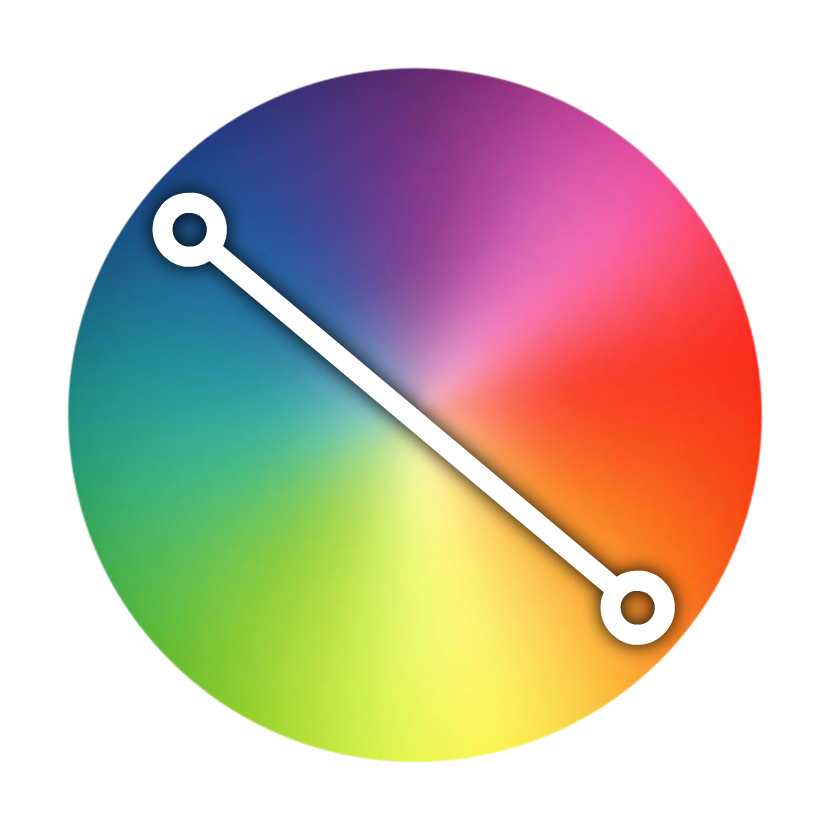For example, the complement of the green-blue color is the red-orange. To put it more generically and mathematically, the complement of a color is always going to be 180 degrees away. This means, our code for picking a complementary color based on a primary color can look as follows:

``````let h_range = [0, 360];
let s_range = [90, 100];
let l_range = [50, 95];
let a_range = [1, 1];

let color = getRandomColor(h_range, s_range, l_range, a_range);
square.style.backgroundColor = color.hslaValue;

let complementaryColor = getHSLAColor(color.h - 180, color.s, 25, 1);
container.style.backgroundColor = complementaryColor;``````

The crucial line is the one here where we take our hue value from the square and subtract it by 180:

``let complementaryColor = getHSLAColor(color.h - 180, color.s, 25, 1);``

This ensures that our new randomly generated color is based on the complement of the primary square color we are starting of with. Because of how angles in a circle work, it is ok for us to always subtract 180. If the final angle goes below 0 into negative territory, it will still be in the exact opposite direction of where the primary hue value was. So, it's all cool.

Continuing what we've done several times already, when we animate these two colors where the square is one color and the container is the complement of the square's color, you'll get something that looks as follows (see source):

I will admit. This isn't the most pleasing color combination most of the time, but you get some killer combinations every now and then. The important part is that you can mess with the angle of the hue value to get various color combinations.

## Conclusion

If you thought generating random colors would be easy, well...it sorta kinda is. Like with many things we deal with, the easiness depends on how customizable you want the final outcome to be. We looked at a simple approach that generates a random RGB value without any added fuss. For the ultimate in customizability, we looked at a second approach that uses the more flexible HSLA color value to allow you to tweak every part of what the final color that gets generated. With flexibility does come added complexity, but if you are serious about the randomly generated color that comes out at the end, the added complexity is totally worth it.

Just a final word before we wrap up. If you have a question and/or want to be part of a friendly, collaborative community of over 220k other developers like yourself, post on the forums for a quick response!